## How to list all possible combinations from single column in Excel?

If you want to return all possible combinations from single column data to get the result as below screenshot shown, do you have any quick ways for dealing with this task in Excel?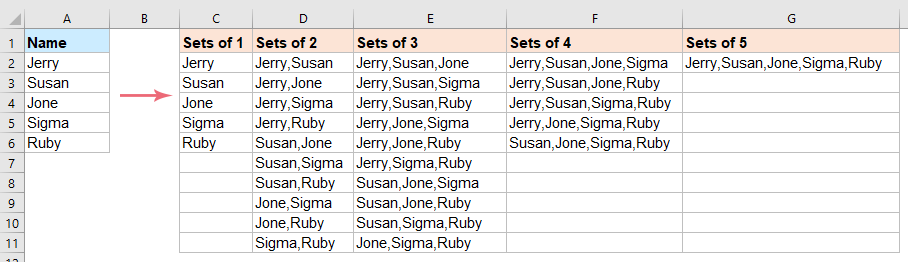List all possible combinations from single column with formulas

List all possible combinations from single column with VBA code

#### List all possible combinations from single column with formulas

1. First, you should create two helper formula cells. In cell C1, please enter the below formula, and press Ctrl + Shift + Enter keys to get the result:

=MAX(LEN(A2:A6))
Note: In this formula, A2:A6 is the list of cells that you want to list their combinations.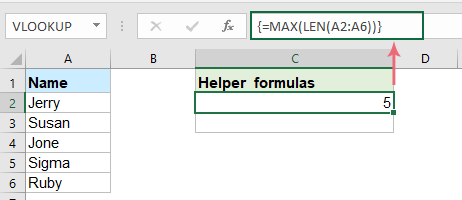2. In cell C2, enter the following formula, and press Ctrl + Shift + Enter keys together to get the second result, see screenshot:

=CONCAT(A2:A6&REPT(" ",C2-LEN(A2:A6)))
Note: In this formula, A2:A6 is the list of cells that you want to list their combinations, C2 is the cell contains the formula you created in step 1.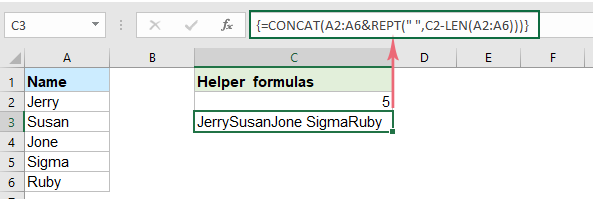3. Then, copy and paste the following formula in cell D2, and press Ctrl + Shift + Enter keys together to get the first result, see screenshot:

=IF(ROW()>2^(COUNTA(A\$2:A\$6)),"",TEXTJOIN(" + ",TRUE,IF(MID(TEXT(DEC2BIN(ROW()-1),REPT("0",COUNTA(\$A\$2:\$A\$6))),ROW(INDIRECT("1:"&COUNTA(\$A\$2:\$A\$6))),1)+0,TRIM(MID(\$C\$3,(ROW(INDIRECT("1:"&COUNTA(\$A\$2:\$A\$6)))-1)*\$C\$2+1,\$C\$2)),"")))
Note: In this formula, A2:A6 is the list of cells that you want to list their combinations, C2 is the cell contains the formula you created in step 1, C3 is the cell with the formula you created in step 2, the + character is the separator to separate the combinations, you can change them to you need.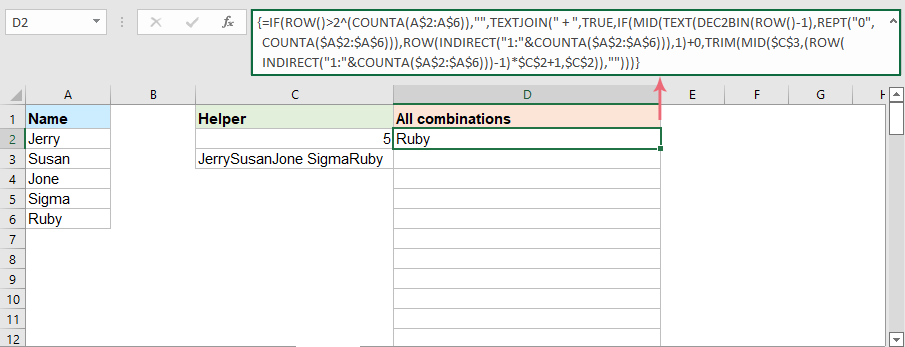4. And then, select this formula cell, and drag the fill handle down until blank cells appear. Now, you can see all combinations of the specified column data are displayed as below demo shown: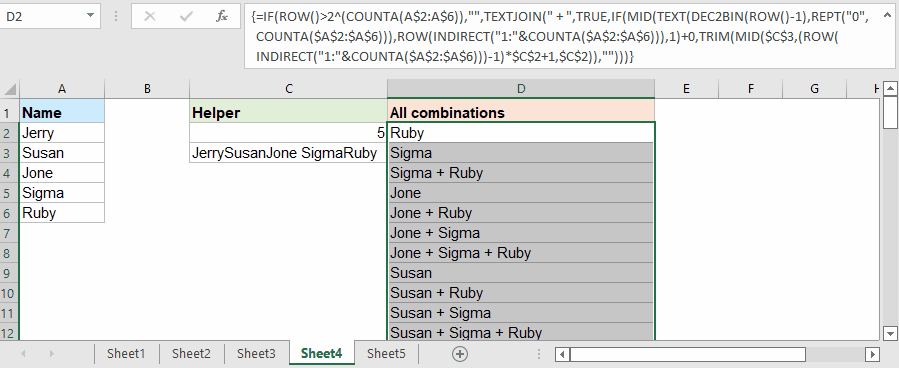Note: This formula is only available in Office 2019, 365 and later version.

#### List all possible combinations from single column with VBA code

The above formulas are only available for newer Excel versions, if you have earlier Excel versions, the following VBA code can do you a favor.

1. Press Alt+ F11 keys simultaneously to open the Microsoft Visual Basic for Applications window.

2. Then, click Insert > Module, copy and paste the below VBA code into the window.

VBA code: List all possible combinations from single column

``````Sub ConnectArr()
'Updateby ExtendOffice
Dim xDValue As Variant
Dim xOutRg As Range
Dim xDictionary As Object
Dim xF As Long
Dim xChar As String
xDValue = Range("A2:A6").Value 'the data range
Set xOutRg = Range("C1") 'output range
xChar = "," 'separator
For xF = 1 To UBound(xDValue)
Set xDictionary = CreateObject("Scripting.Dictionary")
xDictionary(0) = "Sets of " & xF
Call ConnectValue(xDValue, xDictionary, 0, xF, 0, "", xChar)
xOutRg.Offset(0, xF - 1).Resize(xDictionary.Count).Value = WorksheetFunction.Transpose(xDictionary.Items)
Set xDictionary = Nothing
Next
End Sub
Sub ConnectValue(ByRef pDValue, ByRef pDictionary, ByRef pLevel, ByVal pMaxLevel, ByVal pIndex, ByVal pValue, ByVal pChar)
Dim xF As Long
If pLevel = pMaxLevel Then
pDictionary(pDictionary.Count + 1) = pValue
Exit Sub
End If
For xF = pIndex + 1 To UBound(pDValue)
If pValue = "" Then
Call ConnectValue(pDValue, pDictionary, pLevel + 1, pMaxLevel, xF, pDValue(xF, 1), pChar)
Else
Call ConnectValue(pDValue, pDictionary, pLevel + 1, pMaxLevel, xF, pValue & pChar & pDValue(xF, 1), pChar)
End If
Next
End Sub
``````
Note: In the above code:
• A2:A6: is the list of data that you want to use;
• C1: is the output cell;
• ,: the delimiter to separate the combinations.

3. And Then, press F5 key to execute this code. All combinations from the single column are listed as below screenshot shown:### Best Office Productivity Tools

Supercharge Your Spreadsheets： Experience Efficiency Like Never Before with Kutools for Excel

 Popular Features: Find/Highlight/Identify Duplicates   |  Delete Blank Rows   |  Combine Columns or Cells without Losing Data   |   Round without Formula ... Super Lookup: Multiple Criteria VLookup  |   Multiple Value VLookup  |   VLookup Across Multiple Sheets   |   Fuzzy Lookup .... Advanced Drop-down List: Quickly Create Drop Down List   |  Dependent Drop Down List   |  Multi-select Drop Down List .... Column Manager: Add a Specific Number of Columns   |   Move Columns   |   Unhide Columns   |   Compare Columns to Select Same & Different Cells ... Featured Features: Grid Focus   |  Design View   |   Big Formula Bar   |  Workbook & Sheet Manager   |  Resource Library (Auto Text)   |  Date Picker   |  Combine Worksheets   |  Encrypt/Decrypt Cells   |  Send Emails by List   |  Super Filter   |   Special Filter (filter bold/italic/strikethrough...) ... Top 15 Toolset:  12 Text Tools (Add Text, Remove Characters, ...)   |   50+ Chart Types (Gantt Chart, ...)   |   40+ Practical Formulas (Calculate age based on birthday, ...)   |   19 Insertion Tools (Insert QR Code, Insert Picture from Path, ...)   |   12 Conversion Tools (Numbers to Words, Currency Conversion, ...)   |   7 Merge & Split Tools (Advanced Combine Rows, Split Cells, ...)   |   Many More...

Kutools for Excel boasts over 300 features, ensuring that what you need is just a click away...

Supports Office/Excel 2007-2021 & newer, including 365   |   Available in 44 languages   |   Enjoy a full-featured 30-day free trial.#### Office Tab Brings Tabbed interface to Office, and Make Your Work Much Easier

• Enable tabbed editing and reading in Word, Excel, PowerPoint, Publisher, Access, Visio and Project.
• Open and create multiple documents in new tabs of the same window, rather than in new windows.
• Increases your productivity by 50%, and reduces hundreds of mouse clicks for you every day!No ratings yet. Be the first to rate!
This comment was minimized by the moderator on the site
Hello,
Nice job!
But I'm interested to find just the "Sets of 2", as in your example, e.g. a list of players who have to play matches with each other :).
Thank you.
This comment was minimized by the moderator on the site
Hello, Iulian,
Note: your names should be start at A2 cell, and the result will be placed at C2 cell.
``````Sub name_by_name()
Dim i As Long, j As Long, lr As Long
With ActiveSheet
lr = .Cells(Rows.Count, 1).End(xlUp).Row
For i = 2 To lr
For j = i + 1 To lr
.Cells(Rows.Count, 3).End(xlUp).Offset(1, 0) = _
.Cells(i, 1).Value & ", " & .Cells(j, 1).Value
Next j
Next i
End With
End Sub``````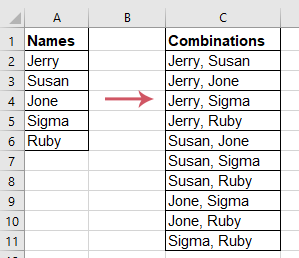This comment was minimized by the moderator on the site
Hello, I have a list of 30 items in a column and the code doesn't seem to be able to handle that, what is the max number of items allowed for the code to work? is there a way to make a long list work?
This comment was minimized by the moderator on the site
Hello, Lynn,
Yes, as you said, if the number of cells are greater than 20, the code will not work well.
Sorry for that inconvenience.

With this code, it will pop out an alert if the number of cells is greater than 20.
``````Sub ConnectArr()
'Updateby ExtendOffice
Dim xDValue As Variant
Dim xOutRg As Range
Dim xDictionary As Object
Dim xF As Long
Dim xChar As String
xAddress = "A1:A20" 'the data range
MsgBox "The number of cells can't greater than 20!"
Exit Sub
End If
Set xOutRg = Range("C1") 'output range
xChar = "," 'separator
For xF = 1 To UBound(xDValue)
Set xDictionary = CreateObject("Scripting.Dictionary")
xDictionary(0) = "Sets of " & xF
Call ConnectValue(xDValue, xDictionary, 0, xF, 0, "", xChar)
xOutRg.Offset(0, xF - 1).Resize(xDictionary.Count).Value = WorksheetFunction.Transpose(xDictionary.Items)
Set xDictionary = Nothing
Next
End Sub
Sub ConnectValue(ByRef pDValue, ByRef pDictionary, ByRef pLevel, ByVal pMaxLevel, ByVal pIndex, ByVal pValue, ByVal pChar)
Dim xF As Long
If pLevel = pMaxLevel Then
pDictionary(pDictionary.Count + 1) = pValue
Exit Sub
End If
For xF = pIndex + 1 To UBound(pDValue)
If pValue = "" Then
Call ConnectValue(pDValue, pDictionary, pLevel + 1, pMaxLevel, xF, pDValue(xF, 1), pChar)
Else
Call ConnectValue(pDValue, pDictionary, pLevel + 1, pMaxLevel, xF, pValue & pChar & pDValue(xF, 1), pChar)
End If
Next
End Sub

``````
This comment was minimized by the moderator on the site
I really like the method but values bottom out at the 511th row and you get #NUM! if you have more than 6 entries in column A. I'm wondering if someone might consider helping me to adjust the formula so that the resulting values calculate beyond the 511th row? Thank you very much! =)
This comment was minimized by the moderator on the site
Hello,
Yes, as you said, the formula will stop work in row 511. So, here, you can appy the VBA code in this article.
Or if you want to list all possible combinations into single one column, please apply the below code:
Note: In the code, A2 is the first cell contains the data you want to use, you should change the cell reference A2 and A to your own. After running the code, all combinations will be listed in the next column of the data list.
``````Sub allcombination()
Dim Ray As Variant, n As Long, nn As Long, Allnum As String, c As Long
Dim Res As Long, obit, oSt, ipc, Tot As Long, oPst As Long, sNum As String
Ray = Application.Transpose(Range(Range("A2"), Range("A" & Rows.Count).End(xlUp)))
sNum = Join(Evaluate("TRANSPOSE(ROW(" & 1 & ":" & UBound(Ray) & "))"), ",")
For n = 1 To UBound(Ray)
Tot = Tot + Application.Combin(UBound(Ray), n)
Next n
ReDim Oval(1 To Tot)
ReDim nRay(1 To Tot - UBound(Ray))
Do Until Allnum = sNum
If c < UBound(Ray) Then
For n = 1 To UBound(Ray)
c = c + 1: Oval(c) = n
Next n
Else
For n = 1 To UBound(Ray)
Res = Res + 1
obit = Oval(Res)
oSt = Split(obit, ",")(UBound(Split(obit, ",")))
For nn = oSt + 1 To UBound(Ray)
c = c + 1
Allnum = obit & "," & nn
Oval(c) = Allnum
Next nn
Next n
End If
Loop
Dim s As Variant, nStr As String
For oPst = UBound(Ray) + 1 To UBound(Oval)
For Each s In Split(Oval(oPst), ",")
nStr = nStr & IIf(nStr = "", Ray(s), "," & Ray(s))
Next s
nRay(oPst - UBound(Ray)) = nStr: nStr = ""
Next oPst
Range("B1").Resize(UBound(nRay)).Value = Application.Transpose(nRay)
End Sub
``````

This comment was minimized by the moderator on the site
Dear skyyang:

Thank you very much for your help and the code. It's invaluable and I'm grateful.

I'm relatively new to VB scripting, consequently not very adept at coding the language.

Just a point or two:

- Your suggested code doesn't generate single entries (e.g. Ruby, or...)
- The original ordering as highlighted in the animated graphic in Step 4 disappeared.

I will go through your code to try my hand at calibrating it so that the above points are outputted, but I was wondering if you had any quick advice or suggestion(s) that could address them.

Thank you again for your kind help. I really appreciate it. =)

My best.
This comment was minimized by the moderator on the site
Dear skyyang:

First, thank you very much for your code solution. I am grateful! =)

I wrote a reply yesterday but the system seems not to have posted it for unknown reasons. I hope this one gets through.

Your code generates output that I am interested in. I had just a couple of observations and then a question:

1) The code doesn't generate the individual entries alone.
2) The original ordering seen in the animated graphic in Step 4 is lost.

From your code is there a way to also include the single entries and to mirror the original ordering format from Step 4. I'm rather new to VB scripting.

Again, thank you so much for your invaluable help. I really appreciate it.

My best.
This comment was minimized by the moderator on the site
Dear skyyang:

This is wonderful. Thank you, this helps me out immensely. I am very grateful.

Just a couple observations I noticed after generating the VB code you provided was that the singletons (for lack of a better term), like just "Ruby", would get omitted, and the resulting (columnal) ordering no longer corresponded to the original ordering generated in Step 4 animated graphic.

Do you happen to have any quick suggestions about how I could tweak your code to also include the "singletons" and for matching the same ordering as in Step 4? I will try to wrangle the workaround but regrettably I'm fairly new to VB scripting.

Thanks again! I really appreciate it.

My best. =)
This comment was minimized by the moderator on the site
Hello, ffuuzz
In this case, you can try the vba code in our article, all possible combinations will be listed into separated columns, please try:
``````Sub ConnectArr()
'Updateby ExtendOffice
Dim xDValue As Variant
Dim xOutRg As Range
Dim xDictionary As Object
Dim xF As Long
Dim xChar As String
xDValue = Range("A2:A6").Value 'the data range
Set xOutRg = Range("C1") 'output range
xChar = "," 'separator
For xF = 1 To UBound(xDValue)
Set xDictionary = CreateObject("Scripting.Dictionary")
xDictionary(0) = "Sets of " & xF
Call ConnectValue(xDValue, xDictionary, 0, xF, 0, "", xChar)
xOutRg.Offset(0, xF - 1).Resize(xDictionary.Count).Value = WorksheetFunction.Transpose(xDictionary.Items)
Set xDictionary = Nothing
Next
End Sub
Sub ConnectValue(ByRef pDValue, ByRef pDictionary, ByRef pLevel, ByVal pMaxLevel, ByVal pIndex, ByVal pValue, ByVal pChar)
Dim xF As Long
If pLevel = pMaxLevel Then
pDictionary(pDictionary.Count + 1) = pValue
Exit Sub
End If
For xF = pIndex + 1 To UBound(pDValue)
If pValue = "" Then
Call ConnectValue(pDValue, pDictionary, pLevel + 1, pMaxLevel, xF, pDValue(xF, 1), pChar)
Else
Call ConnectValue(pDValue, pDictionary, pLevel + 1, pMaxLevel, xF, pValue & pChar & pDValue(xF, 1), pChar)
End If
Next
End Sub
``````
There are no comments posted here yet